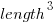## Dimensional analysis of the Halstead metrics

One of the driving forces behind the Halstead complexity metrics was physics envy; the early reports by Halstead use the terms software physics and software science.

One very simple, and effective technique used by scientists and engineers to check whether an equation makes sense, is dimensional analysis. The basic idea is that when performing an operation between two variables, their measurement units must be consistent; for instance, two lengths can be added, but a length and a time cannot be added (a length can be divided by time, returning distance traveled per unit time, i.e., velocity).

Let’s run a dimensional analysis check on the Halstead equations.

The input variables to the Halstead metrics are:, the number of distinct operators,, the number of distinct operands,, the total number of operators, and, the total number of operands. These quantities can be interpreted as units of measurement in tokens.

The formula are:

• Program length:There is a consistent interpretation of this equation: operators and operands are both kinds of tokens, and number of tokens can be interpreted as a length.
• Calculated program length:There is a consistent interpretation of this equation: the operand of a logarithm has to be dimensionless, and the convention is to treat the operand as a ratio (if no denominator is specified, the value 1 is taken), the value returned is dimensionless, which can be multiplied by a variable having any kind of dimension; so again two (token) lengths are being added.
• Volume:A volume has units of(i.e., it is created by multiplying three lengths). There is only one length in this equation; the equation is misnamed, it is a length.
• Difficulty:Here the dimensions ofandcancel, leaving the dimensions of(a length); now Halstead is interpreting length as a difficulty unit (whatever that might be).
• Effort:This equation multiplies two variables, both having a length dimension; the result should be interpreted as an area. In physics work is force times distance, and power is work per unit time; the term effort is not defined.

Halstead is claiming that a single dimension, program length, contains so much unique information that it can be used as a measure of a variety of disparate quantities.

Halsteadâ€™s colleagues at Purdue were rather damming in their analysis of these metrics. Their report Software Science Revisited: A Critical Analysis of the Theory and Its Empirical Support points out the lack of any theoretical foundation for some of the equations, that the analysis of the data was weak and that a more thorough analysis suggests theory and data donâ€™t agree.

I pointed out in an earlier post, that people use Halstead’s metrics because everybody else does. This post is unlikely to change existing herd behavior, but it gives me another page to point people at, when people ask why I laugh at their use of these metrics.